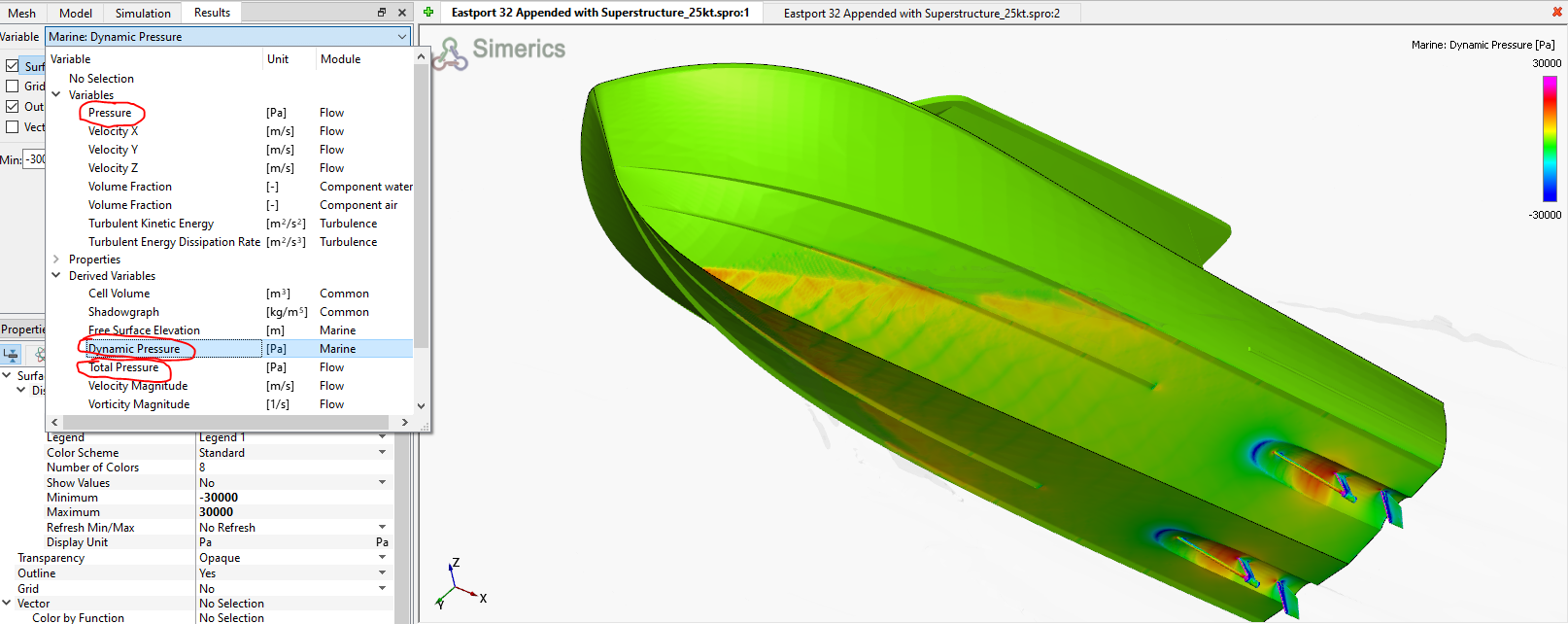As shown in the image below, when plotting pressure distributions in the 3D graphic views, there are 3 pressure variables available. "Pressure" from the Flow module under Variables is the pressure you would measure with a pressure tap, sometimes called gauge pressure. In OMCFD atmospheric pressure is defined as zero so the Flow module Pressure is the pure fluid pressure (dynamic plus hydrostatic).

"Dynamic Pressure" from the Marine module under Derived Variables is defined as the (gauge) Pressure minus the hydrostatic pressure. The Marine module definition of Dynamic Pressure can be both positive and negative (suction). The hydrostatic pressure is referenced to the initial static waterplane. In other words the dynamic pressure is the non-hydrostatic fluid pressure.

"Total Pressure" from the Flow module under Derived Variables is defined as the (gauge) Pressure + 0.5 * rho * V^2. Although perhaps of limited applicability in marine CFD, it can be relevant in other flow problems like internal pump flows. In the case of OMCFD, the reference for velocity here is the inertial coordinate system so the vessel is assumed to be moving at the forward velocity that you can plot.

If you are interested in the absolute pressure, add 1 atmosphere to the Pressure from the Flow module (under Variables). This will then include atmospheric pressure, hydrostatic pressure, and dynamic pressure.

To look for pressure that is low enough for water to flash to vapor, you would plot the Pressure from the Flow module under Variables. If the vapor pressure at the temperature of interest was 2290 Pa and the atmospheric pressure is 101325 Pa, you would look for Pressure that was below (2290-101325)= -99035 Pa.